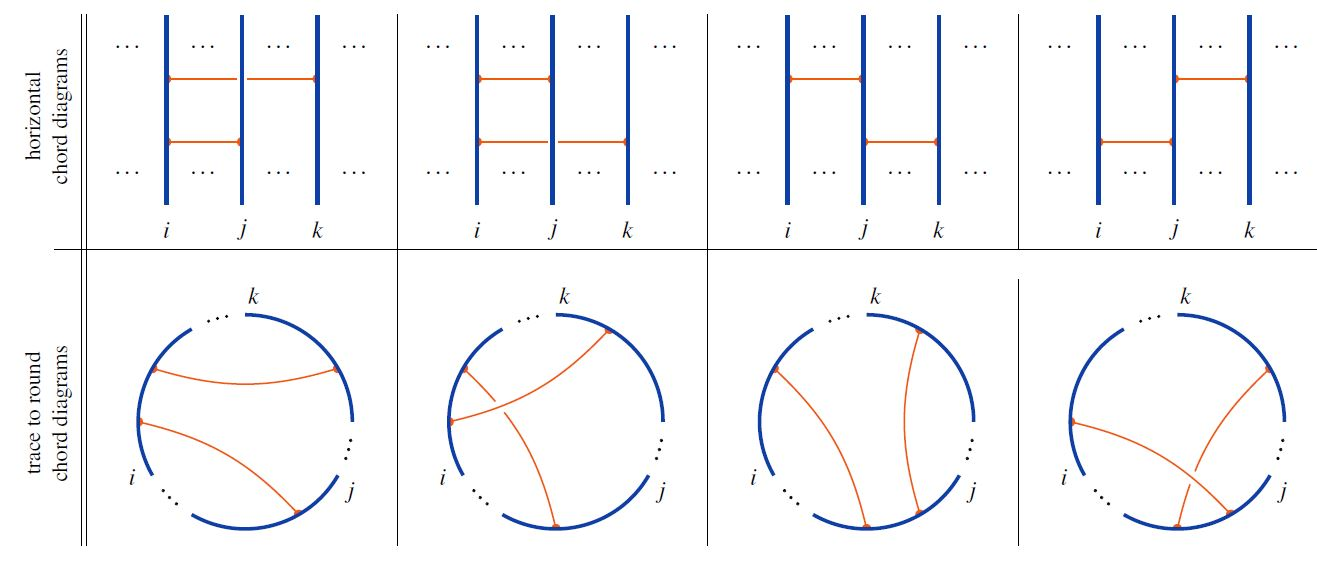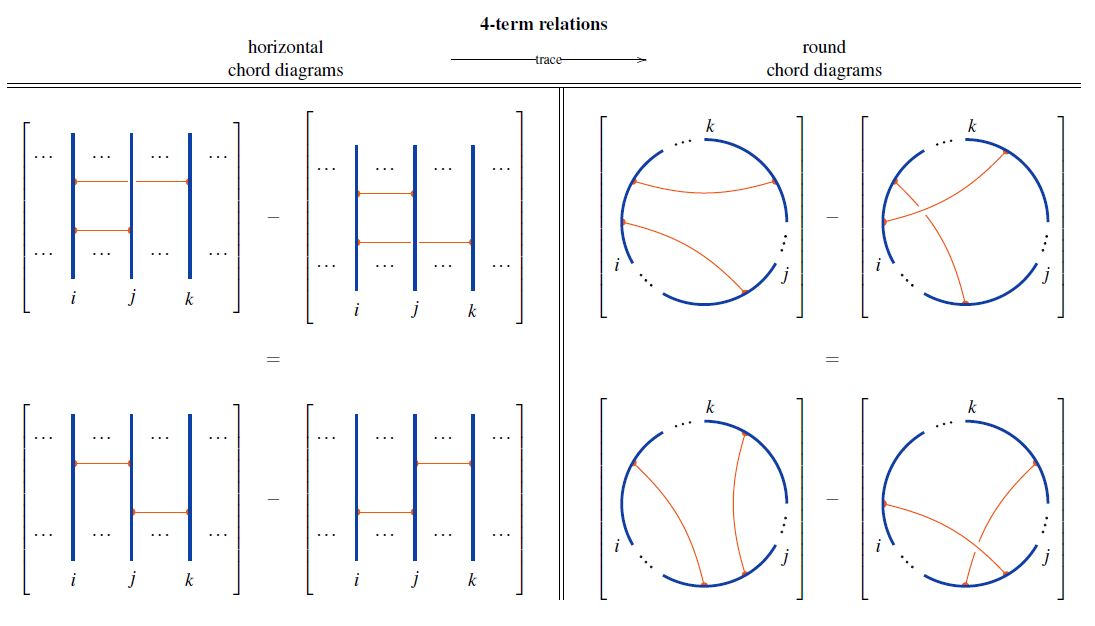# nLab trace of horizontal to round chord diagrams

Contents

### Context

#### Knot theory

knot theory

Examples/classes:

Types

knot invariants

Related concepts:

category: knot theory

# Contents

## Idea

In knot theory, a certain trace-like operation turns horizontal chord diagrams into round chord diagrams:

Given a horizontal chord diagram on $n$ strands and given any choice of cyclic permutation of $n$ elements, the trace is the round chord diagram obtained by gluing the ends of the strands according to the cyclic permutation, and retaining the chords in the evident way.

The following graphics shows basic examples of the trace operation for cyclic permutation of strands one step to the right:## Properties

### Relation to 4T relations

The trace operation sends the 4T relations on horizontal chord diagrams to those for round chord diagrams:The trace operation is implicit in

It is made a little more explicit in